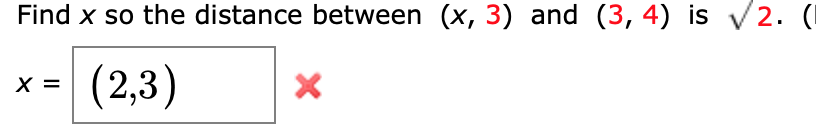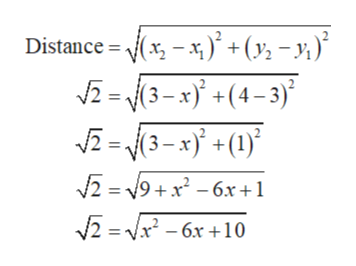# 2. (Find x so the distance between (x, 3) and (3, 4) is(2,3)X

Question
1 views

how do i find the distance between these two pointshelp_outlineImage Transcriptionclose2. ( Find x so the distance between (x, 3) and (3, 4) is (2,3) X fullscreen
check_circle

Step 1

The given points are (x, 3) and (3, 4).

The distance between the points is √2.

Use ...help_outlineImage TranscriptioncloseDistance x + (y, - y;)* 2= 3-x+(4-3) 2= 3-x(1) 2 9+x6x+1 2 x6x10 fullscreen

### Want to see the full answer?

See Solution

#### Want to see this answer and more?

Solutions are written by subject experts who are available 24/7. Questions are typically answered within 1 hour.*

See Solution
*Response times may vary by subject and question.
Tagged in

### Other